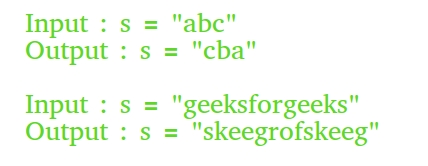Related Articles
Different methods to reverse a string in C/C++
• Difficulty Level : Easy
• Last Updated : 17 Feb, 2021

Given a string, write a C/C++ program to reverse it.1. Write own reverse function by swapping characters: One simple solution is to write our own reverse function to reverse a string in C++.
 `// A Simple C++ program to reverse a string``#include ``using` `namespace` `std;`` ` `// Function to reverse a string``void` `reverseStr(string& str)``{``    ``int` `n = str.length();`` ` `    ``// Swap character starting from two``    ``// corners``    ``for` `(``int` `i = 0; i < n / 2; i++)``        ``swap(str[i], str[n - i - 1]);``}`` ` `// Driver program``int` `main()``{``    ``string str = ``"geeksforgeeks"``;``    ``reverseStr(str);``    ``cout << str;``    ``return` `0;``}`

Output :

`skeegrofskeeg`

2. Using inbuilt “reverse” function: There is a direct function in “algorithm” header file for doing reverse that saves our time when programming.
```// Reverses elements in [begin, end]
void reverse (BidirectionalIterator begin,
BidirectionalIterator end);
```
 `// A quickly written program for reversing a string``// using reverse()``#include ``using` `namespace` `std;``int` `main()``{``    ``string str = ``"geeksforgeeks"``;`` ` `    ``// Reverse str[begin..end]``    ``reverse(str.begin(), str.end());`` ` `    ``cout << str;``    ``return` `0;``}`

Output :

`skeegrofskeeg`
3. Only printing reverse:
 `// C++ program to print reverse of a string``#include ``using` `namespace` `std;`` ` `// Function to reverse a string``void` `reverse(string str)``{``   ``for` `(``int` `i=str.length()-1; i>=0; i--)``      ``cout << str[i]; ``}`` ` `// Driver code``int` `main(``void``)``{``    ``string s = ``"GeeksforGeeks"``;``    ``reverse(s);``    ``return` `(0);``}`

Output:

```skeegrofskeeG
```
4. Getting reverse of a const string:
 `// C++ program to get reverse of a const string``#include ``using` `namespace` `std;`` ` `// Function to reverse string and return``// reverse string pointer of that``char``* reverseConstString(``char` `const``* str)``{``    ``// find length of string``    ``int` `n = ``strlen``(str);`` ` `    ``// create a dynamic pointer char array``    ``char` `*rev = ``new` `char``[n+1];`` ` `    ``// copy of string to ptr array``    ``strcpy``(rev, str);`` ` `    ``// Swap character starting from two``    ``// corners``    ``for` `(``int` `i=0, j=n-1; i

Output:

```skeeGrofskeeG
```
5. Reverse string using the constructor : Passing reverse iterators to the constructor returns us a reversed string.
 `// A simple C++ program to reverse string using constructor ``#include  ``using` `namespace` `std; ``int` `main(){`` ` `    ``string str = ``"GeeksforGeeks"``;`` ` `    ``//Use of reverse iterators``    ``string rev = string(str.rbegin(),str.rend());`` ` `    ``cout<

Output:

```skeeGrofskeeG
```

This article is contributed by Priyam kakati, Ranju Kumari, Somesh Awasthi and improved by Supratik Mitra, Lakshay Bansal. If you like GeeksforGeeks and would like to contribute, you can also write an article and mail your article to contribute@geeksforgeeks.org. See your article appearing on the GeeksforGeeks main page and help other Geeks.